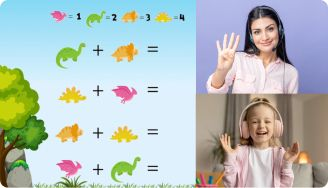# Triangle Inequalities Worksheets

The Triangle Inequality theorem worksheet will help students to learn more about triangle inequality. This theorem states that the sum of the lengths of any two sides of a triangle must be greater than the length of the third side.

Leave your email and we will send you worksheets

worksheets sent successfully

Imagine you have a triangle, and each side is like a rope. The inequality rule says that if you take one rope and add the length of another rope, the total has to be bigger than the length of the third rope. This means that for a triangle with sides of length a, b, and c, the following must be true: a + b > c, b + c > a, and c + a > b.

The theorem applies to all triangles. This is regardless of their shape or size. Before you download our triangle inequality worksheet, let’s look at how triangle inequality works and its be.

## Benefits of Triangle Inequality Worksheets

1:1 Math Lessons

Want to raise a genius?
Start learning Math with BrighterlyAfter studying the triangle inequalities worksheet with answers, students will understand the concept of the triangle equality and be able to solve problems related to it. Other benefits are:

1. Kids can use the knowledge of the triangle inequality theorem to determine whether or not a set of given side lengths can form a valid triangle.
2. This theorem can also be used for problems involving triangles. This includes determining the possible side lengths of a triangle given certain information, or finding missing side lengths in a triangle.

Leave your email and we will send you worksheets

worksheets sent successfully### Triangle Inequalities Worksheets PDF

View worksheet

Triangle Inequality Theorem Worksheet### Triangle Inequalities Worksheets PDF

View worksheet

Worksheet Triangle Inequalities### Triangle Inequalities Worksheets PDF

View worksheet

Triangle Inequality Worksheet### Triangle Inequalities Worksheets PDF

View worksheet

Our triangle inequalities worksheets are free to download and easy for kids to understand. The worksheet triangle inequalities are in PDF formats and printable.

Book 1 to 1 Math Lesson• Specify your child’s math level
• Get practice worksheets for self-paced learning
• Your teacher sets up a personalized math learning plan for your child

Book 1 to 1 Math Lesson